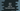# Java program to find the sum of the series 1 + 1/2 + 1/3 + 1/4 or harmonic series## Java program to find the sum of the series 1 + 1/2 + 1/3 + 1/4 + … + 1/n:

In this post, we will learn how to find the sum of the series 1 + 1/2 + 1/3 + 1/4… + 1/n for a given value of n. If you look closely at this series, the inverse numbers in the series follows Arithmetic Progression or A.P.. In Arithmetic progression, if the first value is a and common difference is d, the nth value is a + (n - 1)*d.

Here, the inverse numbers, 1, 2, 3, 4… are following Arithmetic progression, where a = 1 and d = 1.

``````value 1 = a + (1 - 1) * d = 1
value 2 = a + (2 - 1) * d = 1 + 1 = 2
value 3 = a + (3 - 1) * d = 1 + 2*1 = 3
value 4 = a + (4 - 1) * d = 1 + 3*1 = 4
etc..``````

A series with inverse of Arithmetic Progression is called Harmonic series. Here, 1 + 1/2 + 1/3 + 1/4+… is a Harmonic series. The nth value of this series is 1/(a + (n - 1)d).

In this post, we will learn different ways to find the sum of the elements in a Harmonic series upto nth value.

### Method 1: By using a loop:

This is the basic version. We will use one loop to find the sum upto nth value. Below is the complete program:

``````import java.util.Scanner;

class Main {
static double findSum(int n) {
double sum = 0;

for (double i = 1; i <= n; i++) {
sum = sum + 1 / i;
}

return sum;
}

public static void main(String[] args) {
Scanner sc = new Scanner(System.in);
System.out.println("Enter the value of n: ");
int n = sc.nextInt();

System.out.printf("Sum upto " + n + "th value in the series is: %.2f",findSum(n));
}
}``````

Here,

• We are taking the value of n as input from the user and storing it in the variable n.
• findSum method is used to find the sum up to nth place in the harmonic series. It takes the value of n and returns the sum.
• Inside this method, we are using one for loop that runs from i = 1 to i = n. We are simply adding the value of 1/i to sum.
• After the loop ends, sum will hold the required sum value. That is returned from this method.
• We are using printf to print the final sum. It uses .2f to format it to two values after the decimal point.

If you run this program, it will print outputs as like below:

``````Enter the value of n:
6
Sum upto 6th value in the series is: 2.45``````

### Method 2: Recursive approach:

We can solve it in a recursive way. A method is called recursive if it calls itself. We can use a recursive method to find the sum of the above series. below is the complete program:

``````import java.util.Scanner;

class Main {
static double findSum(double n) {
return n == 1 ? 1 : 1/n + findSum(n - 1);
}

public static void main(String[] args) {
Scanner sc = new Scanner(System.in);
System.out.println("Enter the value of n: ");
int n = sc.nextInt();

System.out.printf("Sum upto " + n + "th value in the series is: %.2f",findSum(n));
}
}``````

Here,

• We are using the same method name to find the sum.
• It takes the value of n. It checks if it is equal to 1. If yes, it returns 1, else it returns 1/n + findSum(n - 1). We are using a ternary operator here. It is similar to if-else block, but we can write the if-else condition only in one line.
• i.e. if the value of n is 4,
• first it will call findSum(4), which will return 1/4 + findSum(3), which is 1/4 + 1/3 + findSum(2), which is 1/4 + 1/3 + 1/2+ findSum(1) i.e. 1/4 + 1/3 + 1/2 + 1.

If you run this program, it will print similar output.